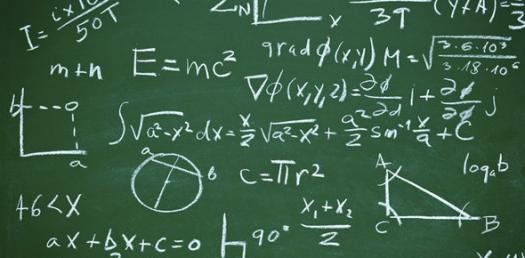# Properties Of Algebra

Approved & Edited by ProProfs Editorial Team
At ProProfs Quizzes, our dedicated in-house team of experts takes pride in their work. With a sharp eye for detail, they meticulously review each quiz. This ensures that every quiz, taken by over 100 million users, meets our standards of accuracy, clarity, and engagement.
| Written by Ahumphreys
A
Ahumphreys
Community Contributor
Quizzes Created: 1 | Total Attempts: 1,269
Questions: 8 | Attempts: 1,297SettingsThis quiz is a review of the properties we have studied this year.

• 1.

### The commutative property works with subtraction.

• A.

True

• B.

False

B. False
Explanation
The commutative property states that the order of the numbers does not affect the result of addition or multiplication. However, it does not hold true for subtraction. The order of the numbers does affect the result of subtraction. For example, 5 - 3 is not the same as 3 - 5. Therefore, the statement "The commutative property works with subtraction" is false.

Rate this question:

• 2.

### Which property is illustrated by the following   (9 + 1) + 4 = 9 + (1 + 4)

• A.

Distributive Property

• B.

• C.

• D.

Explanation
The given equation (9 + 1) + 4 = 9 + (1 + 4) demonstrates the Associative Property of Addition. This property states that the grouping of numbers being added does not affect the sum. In the equation, the numbers are grouped differently on both sides of the equation, but the sum remains the same. This illustrates the associative property, as the grouping of numbers can be changed without changing the result.

Rate this question:

• 3.

### Which of the following operations can be associative?

• A.

Division

• B.

• C.

Subtraction

• D.

Multiplication

D. Multiplication
Explanation
Addition and multiplication can be associative operations. This means that the order in which the operations are performed does not affect the result. For example, (2 + 3) + 4 is equal to 2 + (3 + 4), and (2 * 3) * 4 is equal to 2 * (3 * 4). However, division and subtraction are not associative operations. The order in which these operations are performed does affect the result. Therefore, the correct answer is addition and multiplication.

Rate this question:

• 4.

### What is another word for inverse?

• A.

Random

• B.

Absolute value

• C.

Opposite

C. Opposite
Explanation
Another word for inverse is opposite.

Rate this question:

• 5.

### The following is an example of the associative property of addition:  7 + (3 +4) = (4 + 7) + 3

• A.

True

• B.

False

B. False
Explanation
The given statement is false because it does not represent the associative property of addition. The associative property states that the grouping of numbers being added does not affect the sum. In the given example, the left side of the equation groups 3 and 4 together first, while the right side groups 4 and 7 together first. Therefore, the equation does not demonstrate the associative property and the answer is false.

Rate this question:

• 6.

### Which of the following is an example of the identity property of multiplication:

• A.

7 + 1 = 8

• B.

7 x 1 = 7

• C.

7 + 0 = 7

• D.

7 - 0 = 7

B. 7 x 1 = 7
Explanation
The identity property of multiplication states that any number multiplied by 1 equals the original number. In the given options, the only example that demonstrates this property is 7 x 1 = 7.

Rate this question:

• 7.

### Apply the distributive property to the following expression:   2(9 + 4)

• A.

2 x 13

• B.

2 x 9 + 2 x 4

• C.

2 +9 x 2 + 4

• D.

9 x 4 x 2

B. 2 x 9 + 2 x 4
Explanation
The expression "2(9 + 4)" can be simplified by applying the distributive property. The distributive property states that when a number is multiplied by a sum, it can be distributed to each term within the parentheses. In this case, the number 2 can be distributed to both 9 and 4. Therefore, the expression simplifies to "2 x 9 + 2 x 4". This can be further simplified to "18 + 8", which equals 26.

Rate this question:

• 8.

### Which of the following are NOT the commutative property?

• A.

6 x 7 = 7 x 6

• B.

4 + 9 + 3 = (4 + 9) + 3

• C.

8/3 x 3/8 = 1

B. 4 + 9 + 3 = (4 + 9) + 3
C. 8/3 x 3/8 = 1
Explanation
The commutative property states that the order of the numbers does not affect the result of addition or multiplication. In the given options, both 6 x 7 = 7 x 6 and 8/3 x 3/8 = 1 satisfy the commutative property because the order of the numbers is switched without changing the result. However, 4 + 9 + 3 = (4 + 9) + 3 does not satisfy the commutative property because changing the grouping of the numbers changes the result of the addition. Thus, 4 + 9 + 3 = (4 + 9) + 3 is NOT the commutative property.

Rate this question:

Related TopicsBack to top
×

Wait!
Here's an interesting quiz for you.